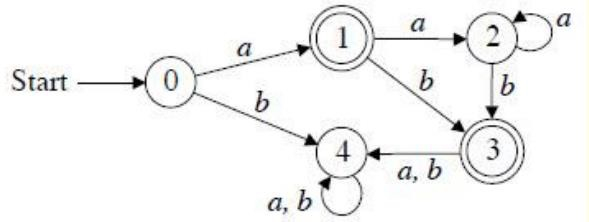# C Program to construct DFA for Regular Expression (a+aa*b)*

Design a Deterministic Finite Automata (DFA) for accepting the language L = (a+aa*b)* If the given string is accepted by DFA, then print “string is accepted”. Otherwise, print “string is rejected”.

## Example 1

Input: Enter Input String
aaaba
Output: String Accepted.

Explanation − The given string is of the form (a+aa*b)* as the first character is a and it is followed by a or ab.

## Example 2

Input: Enter Input String
baabaab
Output: String not Accepted.

The DFA for the given regular expression (a+aa*b) is −Explanation

• If the first character is always a, then traverse the remaining string and check if any of the characters is a or ab.

• If the first character in the string is ‘b’ then it prints “string is rejected”.

• If there exists any character other than a or b while traversing, then it prints “entered value is wrong”.

• Otherwise, print “string is accepted”.

## Program

Following is the C program to construct DFA for Regular Expression (a+aa*b)*

#include<stdio.h>
#include<conio.h>
#include<strings.h>
void main() {
int table,i,j,l,status=0,success;
char input;
printf("To implementing DFA of language (a+aa*b)* Enter Input String:”);
table=1;
table=-1;
table=1;
table=0;
scanf("%s",input);
l=strlen(input);
for (i=0;i<l;i++) {
if(input[i]!='a'&&input[i]!='b') {
printf("The entered Value is wrong");
getch();
exit(0);
}
if(input[i]=='a')
status=table[status]; else
status=table[status];
if(status==-1) {
printf("String not Accepted");
break;
}
}
if(i==l)
printf("String Accepted");
getch();
}

## Output

When the above program is executed, it gives the following output −

Run 1:
To implementing DFA of language (a+aa*b)*
Enter Input String:cbsd
The entered Value is wrong.
Run 2:
To implementing DFA of language (a+aa*b)*
Enter Input String:abbababa
String not Accepted.
Run 3:
To implementing DFA of language (a+aa*b)*
Enter Input String:babbaab
String not Accepted.